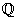index: click on a letter A B C D E F G H I J K L M N O P Q R S T U V W X Y Z A to Z index index: subject areas numbers & symbols sets, logic, proofs geometry algebra trigonometry advanced algebra & pre-calculus calculus advanced topics probability & statistics real world applications multimedia entrieswww.mathwords.com about mathwords website feedback

 Rational Numbers All positive and negative fractions, including integers and so-called improper fractions. Formally, rational numbers are the set of all real numbers that can be written as a ratio of integers with nonzero denominator. Rational numbers are indicated by the symbol. Note: Real numbers that aren't rational are called irrational.See also